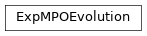# ExpMPOEvolution¶

Inheritance DiagramMethods

 ExpMPOEvolution.__init__(psi, model, options) Initialize self. ExpMPOEvolution.calc_U(dt[, order, …]) Calculate self._U_MPO Run the real-time evolution with the WI/WII approximation. ExpMPOEvolution.update(N_steps) Time evolve by N_steps steps.
class tenpy.algorithms.mpo_evolution.ExpMPOEvolution(psi, model, options)[source]

Bases: object

Time evolution of an MPS using the W_I or W_II approximation for exp(H dt).

[zaletel2015] described a method to obtain MPO approximations $$W_I$$ and $$W_{II}$$ for the exponential U = exp(i H dt) of an MPO H, implemented in make_U_I() and make_U_II(). This class uses it for real-time evolution.

Parameters

Options

config ExpMPOEvolution
option summary

approximation in ExpMPOEvolution.run

Specifies which approximation is applied. The default 'II' is more precise. [...]

combine (from Sweep) in Sweep

Whether to combine legs into pipes. This combines the virtual and [...]

compression_method (from ApplyMPO) in MPO.apply

Mandatory. [...]

dt in ExpMPOEvolution.run

Time step.

init_env_data (from Sweep) in DMRGEngine.init_env

Dictionary as returned by self.env.get_initialization_data() from [...]

lanczos_params (from Sweep) in Sweep

Lanczos parameters as described in [...]

m_temp (from ZipUpApplyMPO) in MPO.apply_zipup

bond dimension will be truncated to m_temp * chi_max

N_steps in ExpMPOEvolution.run

Number of time steps dt to evolve

N_sweeps (from VariationalCompression) in VariationalCompression

Number of sweeps to perform.

order in ExpMPOEvolution.run

Order of the algorithm. The total error scales as O(t*dt^order). [...]

orthogonal_to (from Sweep) in DMRGEngine.init_env

List of other matrix product states to orthogonalize against. [...]

start_env (from Sweep) in DMRGEngine.init_env

Number of sweeps to be performed without optimization to update [...]

start_time

Initial value for :attr:evolved_time.

start_trunc_err

Initial truncation error for :attr:trunc_err

sweep_0 (from Sweep) in Sweep.reset_stats

Number of sweeps that have already been performed.

trunc_params

Truncation parameters as described in :cfg:config:truncate.

trunc_weight (from ZipUpApplyMPO) in MPO.apply_zipup

reduces cut for Schmidt values to trunc_weight * svd_min

verbose (from Sweep) in Sweep

Level of verbosity (i.e. how much status information to print); higher=more [...]

option trunc_params: dict

Truncation parameters as described in truncate.

option start_time: float

Initial value for evolved_time.

option start_trunc_err: TruncationError

Initial truncation error for trunc_err

verbose
Type

int

options

Optional parameters, see run() for more details

Type

Config

evolved_time

Indicating how long psi has been evolved, psi = exp(-i * evolved_time * H) psi(t=0).

Type

float

trunc_err

The error of the represented state which is introduced due to the truncation during the sequence of update steps

Type

TruncationError

psi

The MPS, time evolved in-place.

Type

MPS

model

The model defining the Hamiltonian.

Type

MPOModel

_U

Exponentiated H_MPO;

Type

list of MPO

_U_param

A dictionary containing the information of the latest created _U. We won’t recalculate _U if those parameters didn’t change.

Type

dict

run()[source]

Run the real-time evolution with the WI/WII approximation.

Options

option ExpMPOEvolution.dt: float

Time step.

option ExpMPOEvolution.N_steps: int

Number of time steps dt to evolve

option ExpMPOEvolution.approximation: 'I' or 'II'

Specifies which approximation is applied. The default ‘II’ is more precise. See [zaletel2015] and make_U() for more details.

option ExpMPOEvolution.order: int

Order of the algorithm. The total error scales as O(t*dt^order). Implemented are order = 1 and order = 2.

calc_U(dt, order=2, approximation='II')[source]

Calculate self._U_MPO

This function calculates the approximation U ~= exp(-i dt_ H) with dt_ = dt for order=1, or dt_ = (1 - 1j)/2 dt and dt_ = (1 + 1j)/2 dt for order=2.

Parameters
• dt (float) – Size of the time-step used in calculating _U

• order (int) – 1 or 2

• approximation ('I' or 'II') – Type of approximation for the time evolution operator.

update(N_steps)[source]

Time evolve by N_steps steps.

Parameters

N_steps (int) – The number of time steps psi is evolved by.

Returns

trunc_err – Truncation error induced during the update.

Return type

TruncationError`